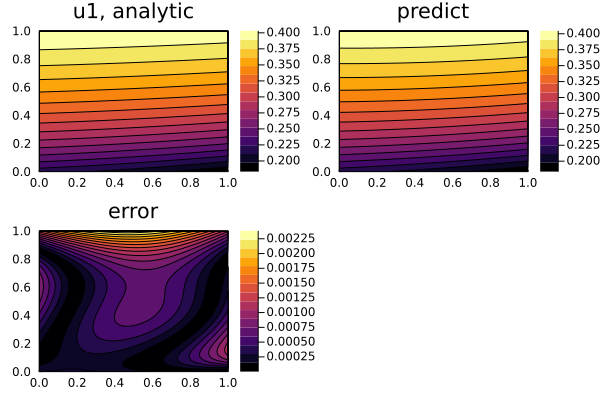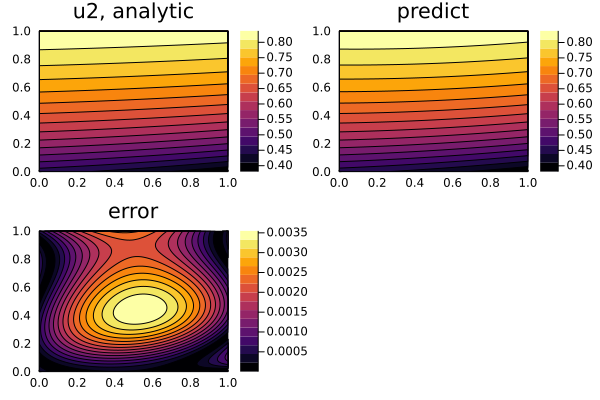# Nonlinear hyperbolic system of PDEs

We may also solve hyperbolic systems like the following

\begin{aligned} \frac{\partial^2u}{\partial t^2} = \frac{a}{x^n} \frac{\partial}{\partial x}(x^n \frac{\partial u}{\partial x}) + u f(\frac{u}{w}) \\ \frac{\partial^2w}{\partial t^2} = \frac{b}{x^n} \frac{\partial}{\partial x}(x^n \frac{\partial u}{\partial x}) + w g(\frac{u}{w}) \\ \end{aligned}

where f and g are arbitrary functions. With initial and boundary conditions:

\begin{aligned} u(0,x) = k * [j0(ξ(0, x)) + y0(ξ(0, x))] \\ u(t,0) = k * [j0(ξ(t, 0)) + y0(ξ(t, 0))] \\ u(t,1) = k * [j0(ξ(t, 1)) + y0(ξ(t, 1))] \\ w(0,x) = j0(ξ(0, x)) + y0(ξ(0, x)) \\ w(t,0) = j0(ξ(t, 0)) + y0(ξ(t, 0)) \\ w(t,1) = j0(ξ(t, 0)) + y0(ξ(t, 0)) \\ \end{aligned}

where k is a root of the algebraic (transcendental) equation f(k) = g(k), j0 and y0 are the Bessel functions, and ξ(t, x) is:

\begin{aligned} \frac{\sqrt[]{f(k)}}{\sqrt[]{\frac{a}{x^n}}}\sqrt[]{\frac{a}{x^n}(t+1)^2 - (x+1)^2} \end{aligned}

We solve this with Neural:

using NeuralPDE, Lux, ModelingToolkit, Optimization, OptimizationOptimJL, Roots
using SpecialFunctions
using Plots
import ModelingToolkit: Interval, infimum, supremum

@parameters t, x
@variables u(..), w(..)
Dx = Differential(x)
Dt = Differential(t)
Dtt = Differential(t)^2

# Constants
a = 16
b = 16
n = 0

# Arbitrary functions
f(x) = x^2
g(x) = 4 * cos(π * x)
root(x) = g(x) - f(x)

# Analytic solution
k = find_zero(root, (0, 1), Roots.Bisection())                # k is a root of the algebraic (transcendental) equation f(x) = g(x)
ξ(t, x) = sqrt(f(k)) / sqrt(a) * sqrt(a * (t + 1)^2 - (x + 1)^2)
θ(t, x) = besselj0(ξ(t, x)) + bessely0(ξ(t, x))                     # Analytical solution to Klein-Gordon equation
w_analytic(t, x) = θ(t, x)
u_analytic(t, x) = k * θ(t, x)

# Nonlinear system of hyperbolic equations
eqs = [Dtt(u(t, x)) ~ a / (x^n) * Dx(x^n * Dx(u(t, x))) + u(t, x) * f(u(t, x) / w(t, x)),
Dtt(w(t, x)) ~ b / (x^n) * Dx(x^n * Dx(w(t, x))) + w(t, x) * g(u(t, x) / w(t, x))]

# Boundary conditions
bcs = [u(0, x) ~ u_analytic(0, x),
w(0, x) ~ w_analytic(0, x),
u(t, 0) ~ u_analytic(t, 0),
w(t, 0) ~ w_analytic(t, 0),
u(t, 1) ~ u_analytic(t, 1),
w(t, 1) ~ w_analytic(t, 1)]

# Space and time domains
domains = [t ∈ Interval(0.0, 1.0),
x ∈ Interval(0.0, 1.0)]

# Neural network
input_ = length(domains)
n = 15
chain = [Lux.Chain(Dense(input_, n, Lux.σ), Dense(n, n, Lux.σ), Dense(n, 1)) for _ in 1:2]

discretization = PhysicsInformedNN(chain, strategy)

@named pdesystem = PDESystem(eqs, bcs, domains, [t,x], [u(t,x),w(t,x)])
prob = discretize(pdesystem, discretization)
sym_prob = symbolic_discretize(pdesystem, discretization)

pde_inner_loss_functions = sym_prob.loss_functions.pde_loss_functions
bcs_inner_loss_functions = sym_prob.loss_functions.bc_loss_functions

callback = function (p, l)
println("loss: ", l)
println("pde_losses: ", map(l_ -> l_(p), pde_inner_loss_functions))
println("bcs_losses: ", map(l_ -> l_(p), bcs_inner_loss_functions))
return false
end

res = Optimization.solve(prob, BFGS(); callback = callback, maxiters=1000)

phi = discretization.phi

# Analysis
ts, xs = [infimum(d.domain):0.01:supremum(d.domain) for d in domains]
depvars = [:u,:w]
minimizers_ = [res.u.depvar[depvars[i]] for i in 1:length(chain)]

analytic_sol_func(t,x) = [u_analytic(t, x), w_analytic(t, x)]
u_real  = [[analytic_sol_func(t, x)[i] for t in ts for x in xs] for i in 1:2]
u_predict  = [[phi[i]([t,x], minimizers_[i]) for t in ts  for x in xs] for i in 1:2]
diff_u = [abs.(u_real[i] .- u_predict[i]) for i in 1:2]
for i in 1:2
p1 = plot(ts, xs, u_real[i], linetype=:contourf, title="u$i, analytic"); p2 = plot(ts, xs, u_predict[i], linetype=:contourf, title="predict"); p3 = plot(ts, xs, diff_u[i], linetype=:contourf, title="error"); plot(p1, p2, p3) savefig("nonlinear_hyperbolic_sol_u$i")
end